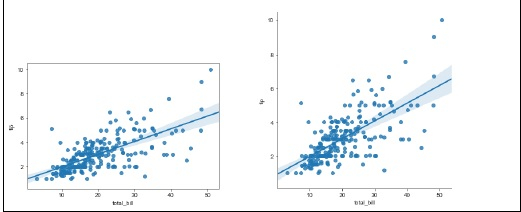# How can a linear relationship be visualized using Seaborn in Python?

Seaborn is a library that helps in visualizing data. It comes with customized themes and a high-level interface.

When regression models are being built, multicollinearity is checked for. This is because we need to understand the correlation present between all different combinations of continuous variables. If multicollinearity exists between the variables, we have to make sure that it is removed from the data. This is where functions ‘regpot’ and ‘implot’ come into play. They help visualize a linear relationship between variables in linear regression.

The ‘regplot’ function accepts values for variables ‘x’ and ‘y’ in a variety of formats, and this includes numpy arrays, pandas series objects, references to variables or values from a pandas dataframe.

On the other hand, the function ‘implot’ requires the user to pass a specific parameter for data, and the values for variables ‘x’ and ‘y’ need to be strings. This type of data format is known as long-form data. Here’s the example −

## Example

import seaborn as sb
from matplotlib import pyplot as plt
sb.regplot(x = "total_bill", y = "tip", data = my_df)
sb.lmplot(x = "total_bill", y = "tip", data = my_df)
plt.show()

## Output## Explanation

• The required packages are imported.
• The input data is ‘tips’ which is loaded from the seaborn library.
• This data is stored in a dataframe.